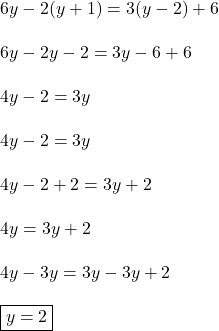## What is the solution to the equation 6y –2(y + 1) = 3(y – 2) + 6? y = –10 y = –2 y = 2 y = 6

Question

What is the solution to the equation 6y –2(y + 1) = 3(y – 2) + 6?
y = –10
y = –2
y = 2
y = 6

in progress 0
1 month 2021-08-02T08:22:21+00:00 2 Answers 3 views 0

1. 6y –2(y + 1) = 3(y – 2) + 6?

The answer is y = 2 (the third option.)